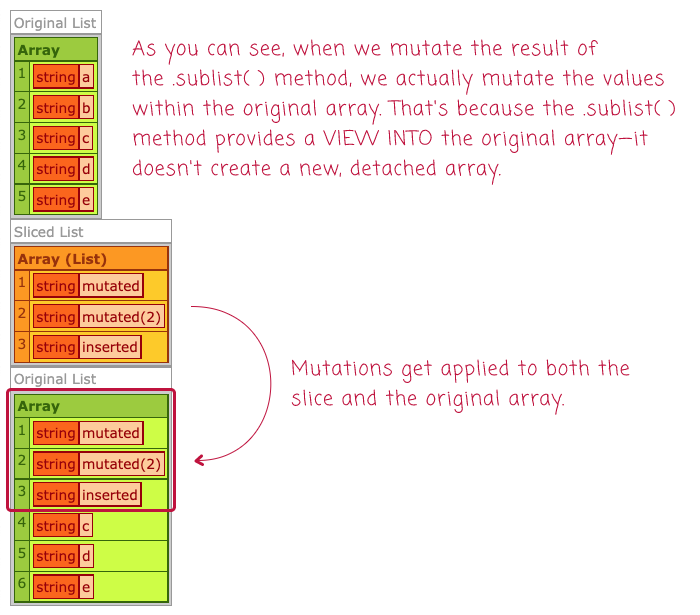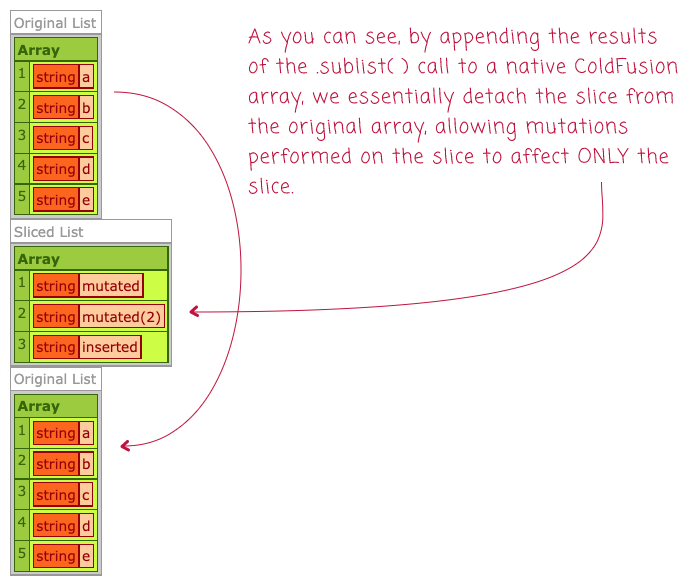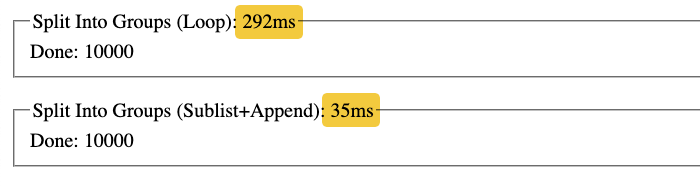# Safely Using Array.sublist() To Generate Slices In Lucee CFML

By on
Tags:

The other day, in the comments of my post on the performance overhead of `arraySlice()` in Lucee CFML, Brad Wood mentioned that it would be much faster to dip down into the Java layer and use `ArrayList.sublist()`. But then, in the comments of the Lucee Jira ticket, Pothys Ravichandran cautioned that `.sublist()` actually returns a wrapper to the original array, not a new array. As such, mutating the results of the `.sublist()` call would not be safe. That said, we can easily generate a new ColdFusion array from the `.sublist()` result in Lucee CFML to keep things running smoothly.

First, let's just quickly demonstrate that `Array.sublist()` is not safe to use (unless you are using it as an internal implementation detail where you know exactly how it is generated and how the result is used). In the following ColdFusion snippet, we're going to use `.sublist()` to access and mutate a slice of an array. And, demonstrate that the mutation to the slice effects the original array as well:

``````<cfscript>

values = [ "a", "b", "c", "d", "e" ];

// Let's get a look at these values BEFORE we start messing with subList().
dump( var = values, label = "Original List" );

// Get a slice of the original array using the Java .sublist() method. The Java
// methods are zero-based (from inclusive, to exclusive).
slicedValues = values.sublist( 0, 2 );
// But, once they are in ColdFusion, they are 1-based.
slicedValues[ 1 ] = "mutated";
slicedValues[ 2 ] = "mutated(2)";
slicedValues.append( "inserted" );

// Let's look at how the mutation above effected BOTH arrays.
dump( var = slicedValues, label = "Sliced List" );
dump( var = values, label = "Original List" );

</cfscript>
``````

When we run this in Lucee CFML 5.3.8.201, we get the following:As you can see, the mutations that we applied to the `.sublist()` array were also applied to the original array. This is because `.sublist()` creates a view into the original array - it does not create a detached array.

To safely use the `Array.sublist()` function, all we have to do is take the result and append it to a native ColdFusion array. In the following ColdFusion code, we are going to use `arrayAppend()` with the `true` flag, which means "append all" values in the given array:

``````<cfscript>

values = [ "a", "b", "c", "d", "e" ];

// Let's get a look at these values BEFORE we start messing with subList().
dump( var = values, label = "Original List" );

// In this version, we're going to use sublist() to LOCATE the values that we want;
// but then, we're going to APPEND() those values into a new ColdFusion array so that
// we can create an independent copy of values, detached from the original array.
// --
// The Java methods are zero-based (from inclusive, to exclusive).
slicedValues = [].append( values.sublist( 0, 2 ), true );

// But, once they are in a ColdFusion array, they are 1-based.
slicedValues[ 1 ] = "mutated";
slicedValues[ 2 ] = "mutated(2)";
slicedValues.append( "inserted" );

// Let's look at IF the mutation above effected BOTH arrays (it does not).
dump( var = slicedValues, label = "Sliced List" );
dump( var = values, label = "Original List" );

</cfscript>
``````

The key line of code here is:

`slicedValues = [].append( SUBLIST, true );`

This creates a new native ColdFusion array and appends all of the values in the `.sublist()` slice to the native ColdFusion array, essentially creating a copy of it. This way, the subsequent mutations get applied to the copy, and not to the original array:Given the fact that the `Array.sublist()` method is both undocumented and tricky to get right, it might be worth avoiding it altogether. That said, it is hella fast! As such, it can be a valuable tool in an algorithm that has to perform a high volume of calculations over a massive array; just like I had to do in my previous post.

When I was looking at how to create a large number of array slices, the fastest approach that I came up with was using the `CFLoop` tag and iteratively building the slices one index at a time. The following snippet is from my previous post and takes an array of 1,000,000 items and splits it up into groups of 100:

``````<cfscript>

include "./utilities.cfm";

// ------------------------------------------------------------------------------- //
// ------------------------------------------------------------------------------- //

things = getRange( 1000000 );

timer
type = "outline"
label = "Split Into Groups (Loop)"
{

groupedThings = splitArrayIntoGroups( things, 100 );

echo( "Done: #groupedThings.len()#" );

}

// ------------------------------------------------------------------------------- //
// ------------------------------------------------------------------------------- //

/**
* I split the collection into groups of the given max-length.
*/
public array function splitArrayIntoGroups(
required array collection,
required numeric maxLength
) {

// PERFORMANCE NOTE: This code looks a bit esoteric; but, that's because this
// method is being used to split MASSIVE ARRAYS (2M+ elements) into smaller
// arrays. The code below is an attempt to remove any calls to methods like .len()
var groups = [];
var groupsLength = 0;
var segment = [];
var segmentLength = 0;

loop
index = "local.i"
item = "local.item"
array = collection
{

segment[ ++segmentLength ] = item;

if ( segmentLength == maxLength ) {

groups[ ++groupsLength ] = segment;
segment = [];
segmentLength = 0;

}

}

if ( segmentLength ) {

groups[ ++groupsLength ] = segment;

}

return( groups );

}

</cfscript>
``````

Here's the same algorithm, but using `Array.sublist()` and `arrayAppend()` to generate the groups:

``````<cfscript>

include "./utilities.cfm";

// ------------------------------------------------------------------------------- //
// ------------------------------------------------------------------------------- //

things = getRange( 1000000 );

timer
type = "outline"
label = "Split Into Groups (Sublist+Append)"
{

groupedThings = splitArrayIntoGroups( things, 100 );

echo( "Done: #groupedThings.len()#" );

}

// ------------------------------------------------------------------------------- //
// ------------------------------------------------------------------------------- //

/**
* I split the collection into groups of the given max-length.
*/
public array function splitArrayIntoGroups(
required array collection,
required numeric maxLength
) {

var collectionLength = collection.len();
var groups = [];

for ( var i = 1 ; i <= collectionLength ; i += maxLength ) {

// Since the .sublist() method is a Java-layer method, it uses 0-based
// indices. As such, we have to translate our 1-based ColdFusion indices over
// by 1 in order to access the right slice.
var fromIndex = ( i - 1 );
var toIndexExclusive = min( collectionLength, ( fromIndex + maxLength ) );

groups.append(
// Append the VIEW of the original slice into a native ColdFusion array
// of its own.
[].append( collection.sublist( fromIndex, toIndexExclusive ), true )
);

}

return( groups );

}

</cfscript>
``````

ASIDE: I'm pretty sure that in Adobe ColdFusion 2021, the `[].append()` call throws a syntax error at compile time. In such a case, you'd have to create an intermediary array variable to hold the slice.

If I run these two "grouping" algorithms back to back, I roughly get the following output (each time I run them, there is some variation, but this is basically the right proportions):As you can see, splitting the array of 1,000,000 items up into groups is 8-times faster using the `Array.sublist()` method, even though we have to explicitly detach the sub-list from the original array by using `Array.append(true)`.

Normally our data-sets are so small that an 8x improvement would likely be imperceptible. However, in my case, I was originally operating on an Array that contained 2M items. As such, an 8x performance improvement would have had a meaningful impact on the overall algorithm completion.

As the ColdFusion runtime and native data structures become more robust with each edition of the CFML engine, reaching into the Java layer becomes a less common event. That said, the Java layer will always be somewhat faster as it presents fewer abstractions. In this case, the Java-based `ArrayList.sublist()` method is very fast; but, it comes with some caveats that make it tricky to use. Hopefully, this post demonstrates how to use it safely!

Want to use code from this post? Check out the license.Fast-follow, I'm wondering if it would be helpful for ColdFusion to provide a built-in function, `arrayFrom()` that could take array-like and other iteratable values and turn them into native ColdFusion arrays:

This would make dealing with "untrusted" arrays a bit easier.Any difference if you are using .push (latest version of Lucee and CF2021) instead of .append for your arrays?@Frédéric,

An interesting question. I sort of just assumed that `.push()` is an alias for `.append()`. I haven't really played around with it yet. I'll have to do some digging.